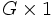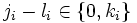# Every finite solvable group is a subgroup of a finite group having subgroups of all orders dividing the group order

This article gives the statement, and possibly proof, of an embeddability theorem: a result that states that any group of a certain kind can be embedded in a group of a more restricted kind.
View a complete list of embeddability theorems

## Statement

Let$G$ be a finite solvable group. Then, there exists a finite group$H$ that is a Group having subgroups of all orders dividing the group order (?), and containing a subgroup isomorphic to$G$.

## Converse

The converse of the statement is true: if a group can be embedded as a subgroup of a finite group having subgroups of all orders dividing the group order, it must be a finite solvable group. This follows from the fact that having subgroups of all orders dividing the group order implies solvable (which in turn follows from Hall's theorem), combined with the fact that solvability is subgroup-closed.

## Facts used

1. ECD condition for pi-subgroups in solvable groups: This is an extended version of Sylow's theorem in finite solvable groups, stating that Hall subgroups of all permissible orders exist.
2. A cyclic group has subgroups of all orders dividing its order.

## Proof

Given: A finite solvable group$G$ of order$p_1^{k_1}p_2^{k_2} \dots p_r^{k_r}$, with$p_i$ prime and$k_i \ge 1$.

To prove: The direct product$H = G \times \mathbb{Z}/m\mathbb{Z}$ has subgroups of all orders dividing its order, where$m = p_1^{k_1 - 1}p_2^{k_2 - 1} \dots p_r^{k_r - 1}$. Note that this direct product contains$G \times 1$, isomorphic to$G$, so this is sufficient.

Proof:$H$ has order:$p_1^{2k_1 - 1}p_2^{2k_2 - 1} \dots p_r^{2k_r - 1}$.

Now, consider any divisor$d$ of the order of$H$, say:$p_1^{j_1}p_2^{j_2} \dots p_r^{j_r}$.

We construct a subgroup of$H$ of this order. First, define$l_i$ as$j_i$ if$j_i < k_i$ and$j_i - k_i$ if$j_i \ge k_i$. Then, find a subgroup$L$ of$\mathbb{Z}/m\mathbb{Z}$ of order:$p_1^{l_1}p_2^{l_2} \dots p_r^{l_r}$.

Now,$j_i - l_i \in \{ 0, k_i \}$, so consider:$p_1^{j_1 - l_1}p_2^{j_2 - l_2} \dots p_r^{j_r - l_r}$.

By fact (1), we conclude that$G$ has a normal subgroup$N$ of this order. Then,$N \times L$ is a subgroup of$H$ of order$d$, as required.